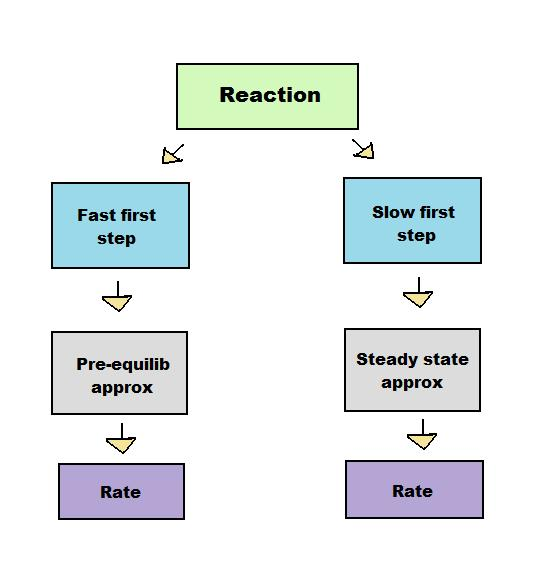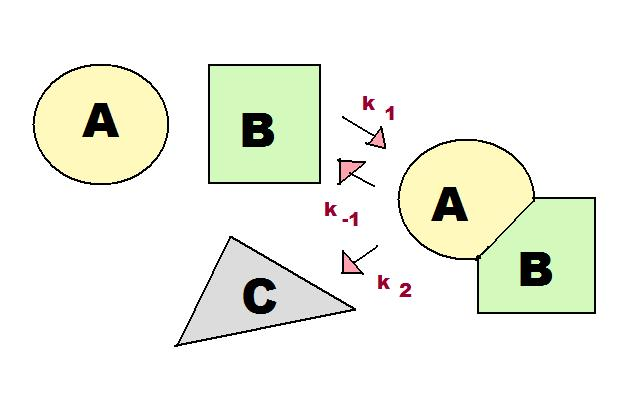# 3.2.2: Pre-equilibrium Approximation

$$\newcommand{\vecs}{\overset { \rightharpoonup} {\mathbf{#1}} }$$ $$\newcommand{\vecd}{\overset{-\!-\!\rightharpoonup}{\vphantom{a}\smash {#1}}}$$$$\newcommand{\id}{\mathrm{id}}$$ $$\newcommand{\Span}{\mathrm{span}}$$ $$\newcommand{\kernel}{\mathrm{null}\,}$$ $$\newcommand{\range}{\mathrm{range}\,}$$ $$\newcommand{\RealPart}{\mathrm{Re}}$$ $$\newcommand{\ImaginaryPart}{\mathrm{Im}}$$ $$\newcommand{\Argument}{\mathrm{Arg}}$$ $$\newcommand{\norm}{\| #1 \|}$$ $$\newcommand{\inner}{\langle #1, #2 \rangle}$$ $$\newcommand{\Span}{\mathrm{span}}$$ $$\newcommand{\id}{\mathrm{id}}$$ $$\newcommand{\Span}{\mathrm{span}}$$ $$\newcommand{\kernel}{\mathrm{null}\,}$$ $$\newcommand{\range}{\mathrm{range}\,}$$ $$\newcommand{\RealPart}{\mathrm{Re}}$$ $$\newcommand{\ImaginaryPart}{\mathrm{Im}}$$ $$\newcommand{\Argument}{\mathrm{Arg}}$$ $$\newcommand{\norm}{\| #1 \|}$$ $$\newcommand{\inner}{\langle #1, #2 \rangle}$$ $$\newcommand{\Span}{\mathrm{span}}$$$$\newcommand{\AA}{\unicode[.8,0]{x212B}}$$

The pre-equilibrium approximation assumes that the reactants and intermediates of a multi-step reaction exist in dynamic equilibrium.

## Introduction

According to the steady state approximation article, reactions involving many steps can be analyzed using approximations. Like the steady state method, the pre-equilibrium approximation method derives an expression for the rate of product formation with approximated concentrations. Unlike the steady state method, the pre-equilibrium approximation does so by assuming that the reactants and intermediate are in equilibrium. Although both methods are used to solve for a rate of reaction, they are used under different conditions. The steady state method can only be used if the first step of a reaction is much slower than the second step, whereas the pre-equilibrium approximation requires the first step to be faster. These opposing conditions prevent the two methods from being interchangeable.Figure 1: The pre-equilibrium approximation is an alternative method to solving for the rate of a reaction, and is used under different conditions than the steady approximation.

Both the steady state approximation and pre-equilibrium approximation apply to intermediate-forming consecutive reactions, in which the product of the first step of the reaction serves as the reactant for the second step.Figure 2. The pre-equilibrium method applies to consecutive reactions where an intermediate is formed. There are three rate constants: $$k_1$$, $$k_{-1}$$, and $$k_2$$. The first two rate constants, $$k_1$$ and $$k_{-1}$$ are used for the reversible steps between reactants ($$A$$ and $$B$$) and intermediate ($$AB$$). $$k_1$$ is used for the forward step and $$k_{-1}$$ is used for the reverse step. $$k_2$$ is the rate constant for the irreversible final step that goes from intermediate ($$AB$$) to product ($$C$$).

As shown in Figure 1, there are three rate constants in the consecutive reaction involving an intermediate. These three rate constants, k1, k-1, and k2, are incredibly important for the pre-equilibrium approximation, as discussed in the next section.

A consecutive reaction found in living systems is the enzyme-substrate reaction. In this type of reaction, an enzyme binds to a substrate to produce an enzyme-substrate intermediate, which then forms the final product. As shown in Figure 2, this reaction follows the basic pattern of the consecutive reaction in Figure 1. The two reactants, E (enzyme) and S (substrate), form the intermediate ES. This enzyme-substrate intermediate forms the product P, usually an essential biomolecule. The enzyme then exits the reaction unchanged and able to catalyze future reactions.

As before, there are three reaction rates in this reaction: k1, k-1, and k2. The pre-equilibrium approximation uses the rate constants to solve for the rate of the reaction, indicating how quickly the reaction is likely to produce the biomolecule.Figure 3. The enzyme-substrate reaction is an important consecutive reaction involved in major biological pathways.

## Derivation of the Rate Law

The enzyme substrate reaction in Figure 2 is used below to demonstrate a rate law derivation. The overall strategy for the pre-equilibrium reaction is as follows:

I. Assume reactants and intermediate are in equilibrium

1. Write out the general reaction
2. Break the overall reaction into elementary steps
3. Assume the reactants and the intermediate are in equilibrium throughout the reaction

II. Solve for the rate of product formation

1. Write out the rate of product formation using the equilibrium constant, K
2. Simplify this rate by incorporating the composite rate constant
3. Simplify to as few variables as possible

I. Assume Reactants and Intermediate are in Equilibrium

1. The general reaction used to derive a rate law is as following:

$$E + S \xrightleftharpoons[k_{-1}]{\ k_1\ } ES \xrightarrow{k_2} E + P$$

2. Breaking up the overall reaction into elementary steps gives:

$E + S \rightarrow ES \nonumber$ Rate of formation of ES = k1 [E][S]

$ES \rightarrow E + S \nonumber$ Rate of decay of ES = k-1 [ES]

$ES \rightarrow E + P \nonumber$ Rate of formation of P = k2 [ES]

3. In the steady state reaction, the intermediate concentration [ES] is assumed to remain at a small constant value. The pre-equilibrium approximation takes a different approach. Because the reversible reaction $$E + S \rightarrow ES$$ is much faster than the product formation of $$ES \rightarrow E + P$$, E, S, and ES are considered to be in equilibrium throughout the reaction. This concept greatly simplifies the mathematics leading to the rate law.

II. Solve for the Rate of Product Formation

4. Using the idea that the reactants and intermediate are in equilibrium, the rate of formation of E + P can be written as:

$\frac{d[P]}{dt}=k_2[ES]=k_2K[E][S] \nonumber$

where

$K = \frac{[ES]}{[E][S]} = \frac{k_1}{k_{-1}} \nonumber$

(K is the equilibrium constant)

5. The rate of product formation is simplified through the composite rate constant, k:

$k = k_2 K =\dfrac{k_1 k_2}{k_{-1}} \nonumber$

6. The rate of product formation can now be shortened to:

$\dfrac{d[P]}{dt} = k[E][S] \nonumber$

This equation can be solved for unknown variables in consecutive reactions involving intermediates.

## Activation Energies in the Pre-equilibrium ReactionFigure 4. Potential Energy (PE) vs Rxn Coordinate for two pre-equilibrium reactions.
Left graph
shows a positive overall activation energy (Ea > 0) and right graph shows a

negative overall activation energy (Ea < 0).

Many reactions have at least one activation energy that must be reached in order for the reaction to go forward. There are three activation energies in a pre-equilibrium reaction: two for the reversible steps and one for the final step. The three activation energies are denoted Ea,1 Ea,-1, and Ea,2:

 Ea,1 Forward Ea,-1 Reverse Ea,2 Product Formation

The overall activation energy is given below:

$E_a= E_a,_1 + E_a,_2 - E_{a, -1} \nonumber$

The overall activation energy is positive if Ea1 + Ea2> Ea-1 The overall activation energy is negative if Ea1 + Ea2 < Ea -1

## Practice Problem

For the reaction $$A + B \xrightarrow[k_{-1}]{k_1} AB \xrightarrow[]{k_2} C$$(see Figure 1), derive a rate law using the pre-equilibrium approximation.

###### Solution

$$\dfrac{d[P]}{dt} = k[A][B]$$ where $$k =\dfrac{k_1 k_2}{k_{-1}}$$